# The Benefits of Using a Modulated DSC Technique

Modulated DSC has significant advantages over conventional DSC in many ways and the number of benefits continues to grow as new applications are explored. These include:

• Separation of complex transitions into more easily interpreted components
• More accurate measurement of the crystallinity of semicrystalline polymers
• Improved resolution without compromising sensitivity
• Improved sensitivity to detect weak transitions
• Thermal conductivity measurement
• Determination of heat flow and heat capacity in a single experiment

Because the technique provides such significant benefits, and because the transitions (phase changes, chemical reactions, etc.) observed in a sample as it is heated are often very complex, the technique of MDSCTM is often assumed to be more complex than it really is.

The advantages of the MDSC™ technique, including more insight into complex transitions, are the result of performing two concurrent, simple experiments, followed by carrying out two simple calculations based on the resulting heat flow signal. The measurements and calculations utilized in the MDSC method are discussed in the article.

## Two Simultaneous Experiments

Conventional DSC determines the variation in heat flow between an inert reference and sample when they are exposed to a linear temperature change. It can also perform heat capacity measurements by determining the variation in heat flow between two experiments involving the exposure of two identical samples of the same mass to two different linear heating rates.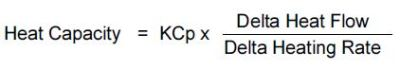Where, KCp = Calibration constant, Delta Heat Flow = Heat flow difference (Exp1 – Exp2), and Delta Heating Rate = Heating Rate Difference (Exp1 – Exp2).

Heat flow and heat capacity can both be measured in a single experiment using the MDSC™ technique by superimposing a modulated heating rate (changing heating rate) over a linear heating rate. An example of the ability to do this is seen in Figure 1 where the average heating rate is 2°C/minute. (linear) while the instantaneous heating rate is changing between a minimum of 0.3°C/minute. and a maximum of 3.7°C/min.. The linear temperature changes allows the heat flow measurement and the modulated change allows the heat capacity calculation.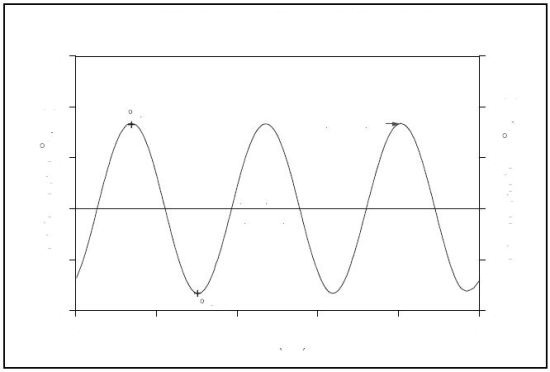Figure 1. MDSC temperature change

## Two Simple Calculations

Figure 2 shows the heat flow results from a linear temperature change (conventional DSC) and Figure 3 shows the modulated heat flow signal that results from the modulated heating rate in MDSC. The benefits of MDSC are obtained from the following two measurements made on the modulated heat flow signal.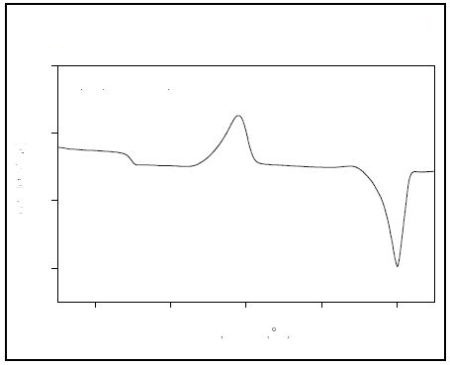Figure 2. Quench cooled PET (Traditional DSC)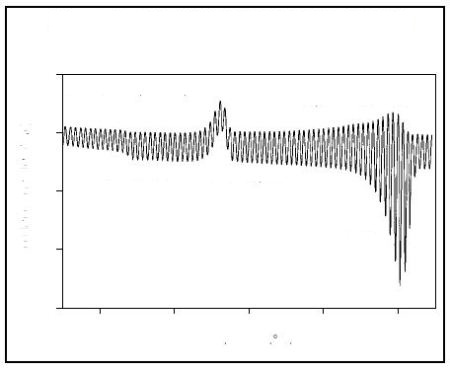Figure 3. Quench cooled PET (MDSC)

## Heat Flow Average

Figure 4 shows the average value of the modulated heat flow signal as the solid line. An analysis of the three transitions reveals that the average value of the modulated heat flow signal is equal to the heat flow signal from conventional DSC both qualitatively and quantitatively, at the same average heating rate. Total heat flow is the name given to this signal (average value) because it consists of the heat flow associated with all thermal events in the sample like the conventional DSC.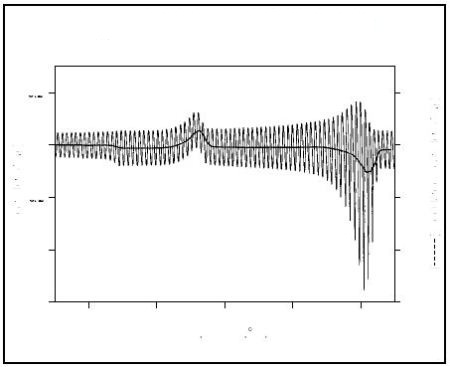Figure 4. The modulated heat flow signal and its average value (solid line)

## Heat Flow Amplitude

The modulated heat flow and modulated heating rate signals are the raw signals generated in an MDSCTM experiment (Figure 5), which calculates the heat capacity using the amplitude of these two signals in the same manner that DSC performs heat capacity calculation from two experiments at different heating rates. However, MDSC calculates heat capacity in a single experiment using a single sample once the instrument has been calibrated.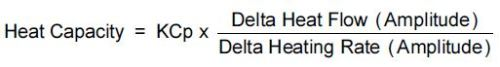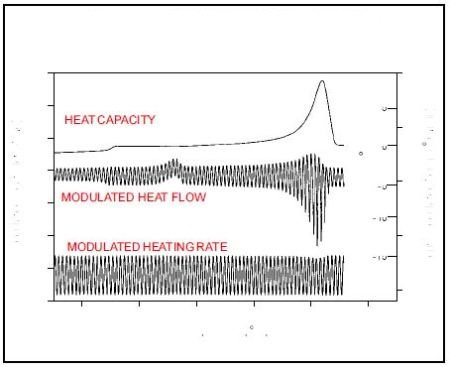Figure 5. The modulated heat flow and modulated heating rate signals are the raw signals created in an MDSC experiment. MDSC uses the amplitude of these two signals to calculate heat capacity.

## Obtaining the Benefits of MDSC

After making two simple measurements (average and amplitude), the benefits of MDSC result from simple arithmetic treatment of the signals. This treatment results in two additional signals.

1. Reversing Heat Flow = Heat Capacity x ( - Average Heating Rate)
2. Nonreversing Heat Flow = Total Heat Flow - Reversing Heat Flow

Reversing Heat Flow is the heat capacity component of the total heat flow. Scaling up this signal to the same heating rate as the total heat flow signal is the reason for multiplying the heat capacity by the average heating rate. This allows subtraction of the two signals to generate the Nonreversing Heat Flow signal, which is the total heat flow signal’s kinetic component. The total heat flow is separated into its reversing and nonreversing components as shown in Figure 6.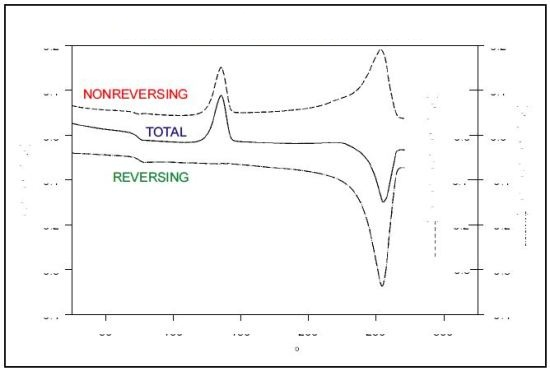Figure 6. The separation of the total heat flow into its reversing and nonreversing components

## Conclusion

Modulated DSC™, which is based on the proven technique of DSC, offers a number of benefits over conventional DSC. It can measure both heat flow and heat capacity by performing two simultaneous experiments. Additionally, the total heat flow can be separated into its heat capacity (reversing) and kinetic (nonreversing) components using simple calculations.This information has been sourced, reviewed and adapted from materials provided by TA Instruments.

## Citations

• APA

TA Instruments. (2020, April 28). The Benefits of Using a Modulated DSC Technique. AZoM. Retrieved on October 27, 2020 from https://www.azom.com/article.aspx?ArticleID=12109.

• MLA

TA Instruments. "The Benefits of Using a Modulated DSC Technique". AZoM. 27 October 2020. <https://www.azom.com/article.aspx?ArticleID=12109>.

• Chicago

TA Instruments. "The Benefits of Using a Modulated DSC Technique". AZoM. https://www.azom.com/article.aspx?ArticleID=12109. (accessed October 27, 2020).

• Harvard

TA Instruments. 2020. The Benefits of Using a Modulated DSC Technique. AZoM, viewed 27 October 2020, https://www.azom.com/article.aspx?ArticleID=12109.